Table of ContentOpen Access

ARTICLE

# Skeleton-Based Volumetric Parameterizations for Lattice Structures

Long Chen1,*, Shuxun Liang2, Nan Yan2, Xiangqian Yang2, Baotong Li3
1 School of Mechanical Engineering, University of Shanghai for Science and Technology, Shanghai, 200093, China
2 School of Optical-Electrical and Computer Engineering, University of Shanghai for Science and Technology, Shanghai, 200093, China
3 Key Laboratory of Education Ministry for Modern Design & Rotor-Bearing System, Xi’an Jiaotong University, Xi’an, 710049, China
* Corresponding Author: Long Chen. Email:(This article belongs to this Special Issue: Integration of Geometric Modeling and Numerical Simulation)

Computer Modeling in Engineering & Sciences 2023, 135(1), 687-709. https://doi.org/10.32604/cmes.2022.021986

Received 16 February 2022; Accepted 23 May 2022; Issue published 29 September 2022

### Abstract

Lattice structures with excellent physical properties have attracted great research interest. In this paper, a novel volume parametric modeling method based on the skeleton model is proposed for the construction of three-dimensional lattice structures. The skeleton model is divided into three types of nodes. And the corresponding algorithms are utilized to construct diverse types of volume parametric nodes. The unit-cell is assembled with distinct nodes according to the geometric features. The final lattice structure is created by the periodic arrangement of unit-cells. Several different types of volume parametric lattice structures are constructed to prove the stability and applicability of the proposed method. The quality is assessed in terms of the value of the Jacobian matrix. Moreover, the volume parametric lattice structures are tested with the isogeometric analysis to verify the feasibility of integration of modeling and simulation.

### Graphical Abstract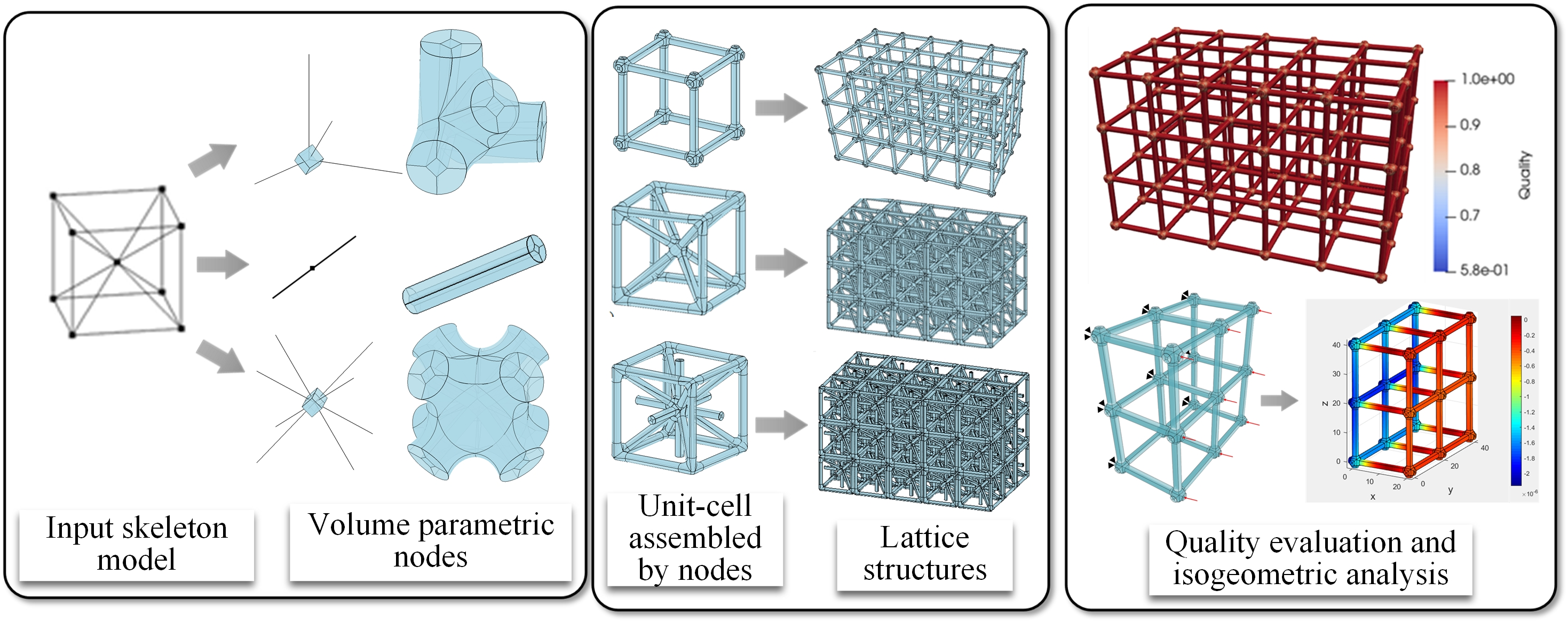### Keywords

Lattice structure; volumetric parameterization; skeleton model; node modeling

1  Introduction

In recent years, the extensive application of additive manufacturing (AM) has rendered possibilities for fabricating complex components, such as lattice structures . The lattice structures exhibit low density, enhanced energy absorption, and excellent mechanical properties . It is widely used in the aerospace, petrochemical, and manufacturing industries as well as other fields . Most researches on lattice structures focus on the boundary representation (B-rep) models, which require to be transformed into a triangular or quadrangular mesh before simulation [6,7]. However, generating the mesh takes 80% of the time in the entire design and analysis process , resulting in a serious reduction in productivity. Therefore, a unified geometric description of modeling and simulation has great advantages. The novel method of isogeometric analysis (IGA)  utilizes the same spline basis functions for geometric modeling and physical simulation making it possible to achieve the goal. Many studies have shown that the IGA has higher accuracy and robustness than the finite element analysis (FEA) . It is of great significance to construct a model suitable for IGA.

In this paper, a volume parametric modeling method of three-dimensional (3D) lattice structures is proposed based on the skeleton. The models constructed by our method are suitable for IGA without any transformation. In the entire modeling process, the construction of the volume parametric nodes is the key step. The unit-cell is assembled with different nodes by permutation and combination. And the lattice structure is created via the periodic arrangement of unit-cells. In addition, the toric surface is used as the transition surface to make the shape of the node smooth, which is beneficial to reduce stress concentration in the analysis. The main contributions are as follows:

1.    A volume parametric modeling method of complex nodes is achieved.

2.    Different types of volume parametric unit-cells and lattice structures are created.

3.    The framework of integration of modeling and simulation is completed.

The remainder of the paper is organized as follows: In Section 2, some backgrounds and related works are introduced. The basic theory and algorithms are explained in Section 3. The modeling method of nodes is described in Section 4. The construction processes of unit-cells and lattice structures are presented in Section 5. Several models are provided to verify the effectiveness and the feasibility of the proposed method in Section 6. Finally, the conclusions are summarized in Section 7.

2  Related Works

The lattice structures with excellent properties have been widely applied in many industries. Researchers have proposed numerous modeling methods to construct lattice structures. Fan et al.  designed a honeycomb lattice structure and a sandwich structure with better mechanical properties than the solid structure, but the model is only two-dimensional. Masalha et al.  studied several algorithms to construct heterogeneous or trivariate fillets that support smooth filleting operation. The results verify the feasibility of the algorithms. Li et al.  proposed an improved algorithm based on the quartet structure generation set method. The algorithm reconstructed the 3D random porous structure. Leblanc et al.  introduced a modeling method based on the block to construct the complex model. The quality of the model heavily relies on the subdivision. Tang et al.  presented a novel design method for the periodic lattice structure. The frame generation algorithm is used to generate lattice wireframes based on the kernel. Tang et al.  studied an innovative hybrid geometric modeling method of lattice structures with three-stage, which involves generating lattice frames, constructing geometric functions, and voxelization. Liu et al.  proposed a memory-efficient modeling method and an adaptive slice algorithm of lattice structure to assist manufacturing. Lattice has an excellent performance in macrostructure as well as in microstructure simulation, such as multiscale crystal defect dynamics . It offers a possible solution to study crystalline plasticity on the nanoscale and mesoscale.

Manufacturing lattice structures is not easy via traditional processing technology due to the complex structure. The development of AM solves this problem. Dong et al.  summarized the existing modeling approaches and the characteristics of different AM in manufacturing cellular materials. Medeiros et al.  proposed an automatic adaptive voids algorithm with AM constraints to fabricate cellular structures with minimal material and maximum strength. Tang et al.  presented a method to connect the design and manufacturing process to improve the stiffness of heterogeneous lattice structures with manufacturing constraints. Garner et al.  utilized the optimization of individual cells and neighboring pairs to find the best connectivity and smoothness of the physical properties among the microstructures. Conde-Rodríguez et al.  proposed a modeling framework of heterogeneous structures based on Bezier patches. The method is appropriate to fabricate structures with different materials, but only single-level details and Bezier were permitted.

Compared with the B-rep models mentioned above, the volume parametric models utilize high-order non-uniform rational B-spline (NURBS) to express the physical domains precisely  and are suitable for IGA without any transformation. Meanwhile, the shape of the model remains unchanged after the refinement. On account of those characteristics, the volume parametric model has attracted a lot of attention. To make use of IGA more convenient, an interactive parametric design-analysis platform is designed . In the meantime, many parametric design methods have been proposed , which greatly extend the geometric modeling method. Moreover, Xiao et al.  utilized the surface interrogation technique for IGA and applied it to large-scale lattice-skin structures of several thousand intersections. Li et al.  investigated the behavior of functionally graded porous plates reinforced by graphene platelets, and incorporated within the IGA framework. Furthermore, different spline basis functions such as T-spline and PHT are utilized to construct the volume parametric model [36,37]. These methods simplify the parameterization process and extend the application of IGA.

In this paper, a volume parametric modeling method of lattice structures based on the skeleton model is proposed. The method solves the problem of hexahedron segmentation of the complex nodes. And the modeling process is simplified by utilizing volume parametric nodes to assemble lattice structures. In addition, the toric surface is used as the transition surface to make the shape of the node smooth. Most importantly, the model constructed by the proposed method is suitable for isogeometric analysis. The proposed method realizes the integration of modeling and simulation.

3  Basic Theories and Overview of the Algorithm

3.1 Representation of the Volumetric Parameterization Model

The physical domain is defined based on the NURBS  and represented by Ω in R3, as shown in Eq. (1).

Ω={x={x,y,z}R3|x=V(u,v,w),0<u,v,w<1}(1)

The volume parametric model and NURBS basis function are described in Eqs. (2) and (3).

V(u,v,w)=i=0lj=0mk=0nRi,j,kp,q,r(u,v,w)Pi,j,k(2)

Ri,j,kp,q,r(u,v,w)=Ni,p(u)Nj,q(v)Nk,r(w)ωi,j,ki=0lj=0mk=0nNi,p(u)Nj,q(v)Nk,r(w)ωi,j,k(3)

where Ri,j,kp,q,r(u,v,w) is the basic function of NURBS, Pi,j,k(i[0,l],j[0,m],k[0,n]) is the control point. Ni,p(u), Nj,q(v) and Nk,r(w) are basis functions of B-spline defined in the three directions U,V,W, respectively. p,q,r are the degrees, ωi,j,k is the weight factor of Pi,j,k.

3.2 Basic Definitions

The skeleton is an abstract description of the geometric model. It is widely used in segmentation, reconstruction, and geometric design. Many extraction algorithms are used to construct the skeleton model . The interactive design is the most commonly used method of skeleton construction . For periodic lattice structures, the unit-cell can be utilized to represent the topological information. In this paper, we build the skeleton model of unit-cells according to the different types of lattice structures and given by interconnected NURBS curves. The relationship of the skeleton is expressed by Eq. (4). Two typical lattice structure skeleton models are shown in Fig. 1.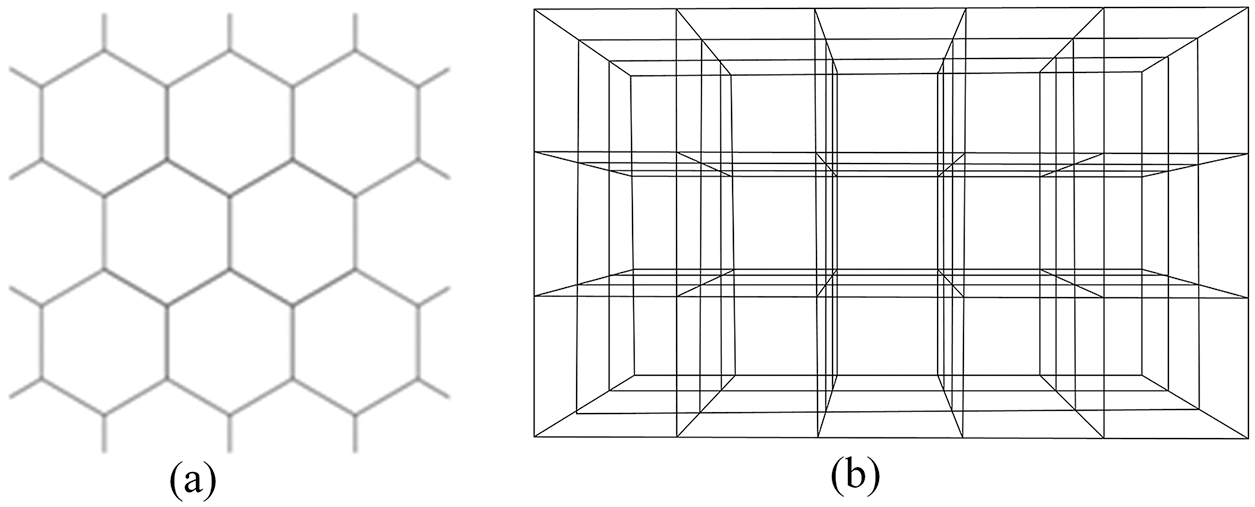Figure 1: Typical skeleton models of the lattice structure. (a) Honeycomb lattice structure; (b) 3D cubic lattice structure

SK={Ci(u)|iZ}(4)

The unit-cell is the basic element to generate a uniform lattice structure. Several representative unit-cells presented by the skeleton models are shown in Fig. 2.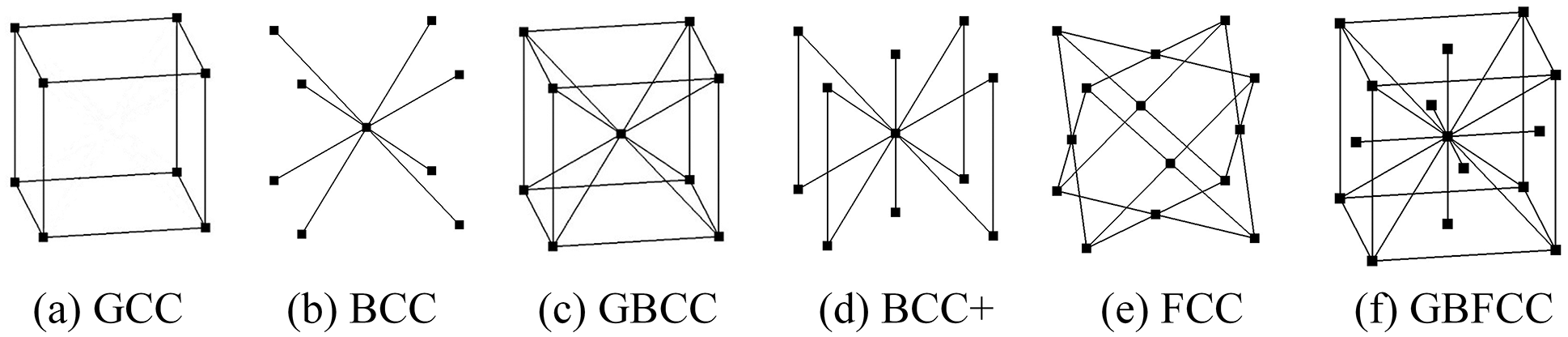Figure 2: Typical unit-cell models obtained using the skeleton model

In this paper, we defined three types of nodes according to the number of branches. One branch indicates the end nodes En, two branches indicate the joint nodes Jn, and others are classified as the branch nodes Bn, as shown in Fig. 3.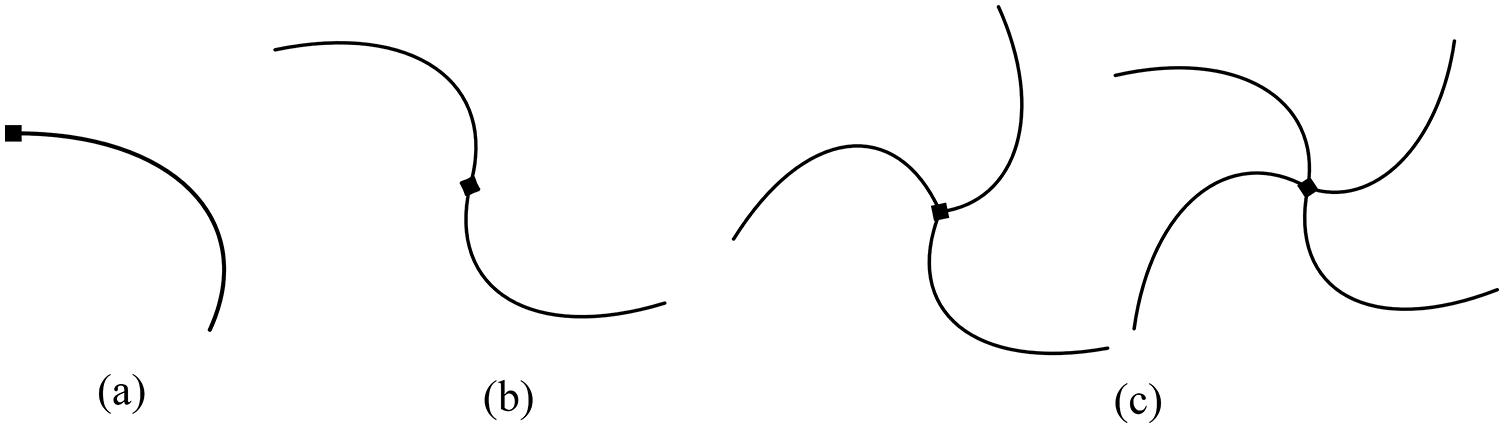Figure 3: Node models obtained with the skeleton curves. (a) En node; (b) Jn node; (c) Bn node

The connection part between nodes is composed of solid cylinders. It is divided into five primitive volumes to satisfy the parameterization requirement, as shown in Fig. 4a. The cross-section of the cylinder is composed of B-spline surfaces Si(i=0,1,2,3,4) with the center point o and radius r. The width of the square is a=r/4. These five surfaces are used to construct the volume parametric models Vi(i=0,1,2,3,4) by sweeping along the skeleton curve, as shown in Fig. 4b.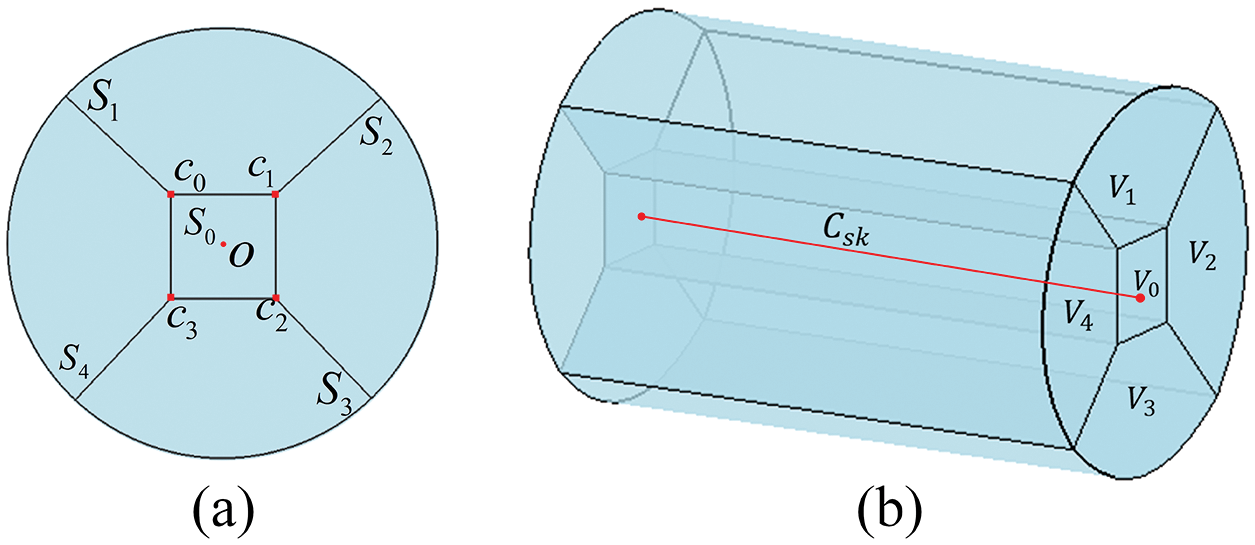Figure 4: The five primitive volumes. (a) Cross-section; (b) volume parametric modeling

3.3 Overview of the Algorithm

The input parameters to the algorithm include the skeleton model Sc and the radius r of the cross-section. The volume parametric lattice structure Vs is the final output. The modeling process is illustrated in Fig. 5. With the designed skeleton model, the nodes are divided into three types namely Bn, Jn, and En. The unit-cell models are assembled with the different nodes according to the geometry and topology information. The volume parametric lattice structure is generated through the periodic arrangement of the unit-cells.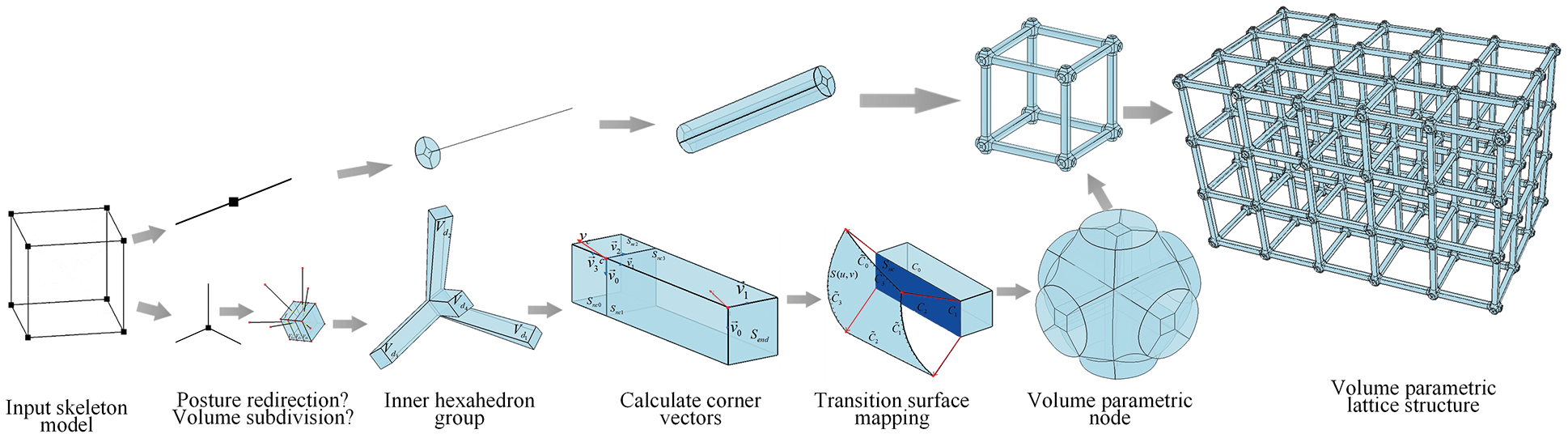Figure 5: Algorithmic flow of the volume parametric lattice structure modeling

4  Modeling of the Nodes

4.1 Inner Hexahedral Group Construction of the Branch Node

Based on the research of the previous work , this study implements a “skeleton-aware” hexahedra group generation method. Firstly, an initial hexahedral box Vb0 of trivariate B-spline volume parallel to the cartesian coordinate axis is generated at the center point of the node. Depending on the tangent direction of the branch curves, Vb0 executes a “posture redirection” operation to make sure the branch curves penetrate from different surfaces. If each surface of Vb0 intersects with only one branch, there is no need to conduct the “volume subdivision” operation. Otherwise, the volume subdivision of Vb0 is executed to generate the final hexahedral box Vbox. Each surface of Vbox can be used to construct a parametric patch along the direction of branch curves. The final hexahedral box of the branch node is shown in Eq. (5), where N is the number of hexahedra.

Vbox={Vb1,,Vbj},jN(5)

The posture redirection operation ensures the branch curves penetrate from different surfaces of Vbox. The above constraint can be converted into the optimization equation shown in Eq. (6). Where d1,..,dk is the direction of the branch curves. The function f(UVW) takes the minimum value. ε is an empirical parameter and greater than zero.

minfε(U,V,W)=j=1k(djU)2+ε+(djV)2+ε+(djW)2+εs.t.{|U|=|V|=|W|=1UW=UV=VW=0ε>0}(6)

This is a nonlinear optimization problem. The length of the orthogonal basis UVW is the constraint. A principal component analysis method is used for d1,,dk to set the initial UVW. The initial value of ε is 0.2 and decreases by a factor of 0.5 in each iteration. The posture of Vb0 is confirmed after five iterations.

Vb0 is updated after the posture redirection operation. As shown in Fig. 6a, the initial hexahedron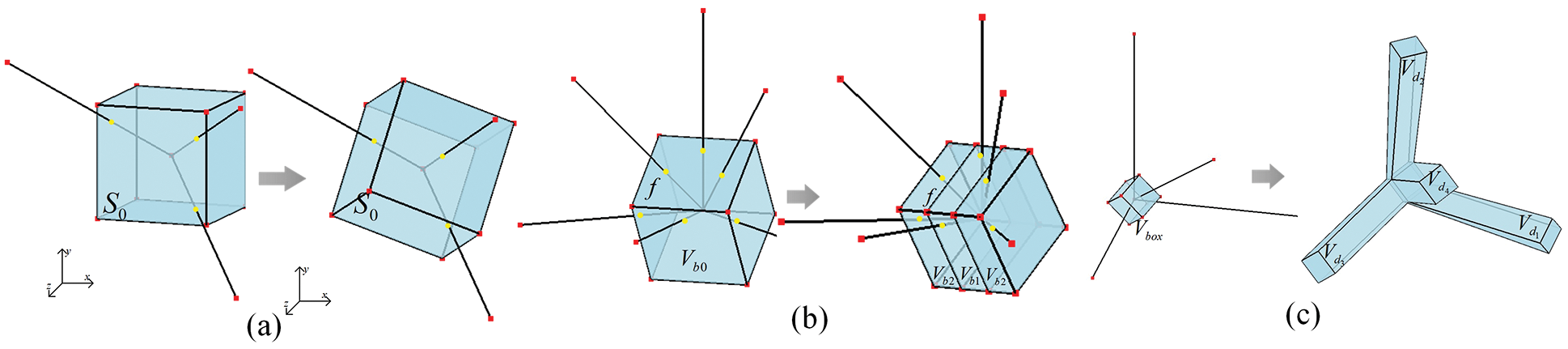Figure 6: Inner hexahedron group generation. (a) Posture redirection operation; (b) volume subdivision operation; (c) branch generation

Vb0 has the three branch curves d1,d2,d3 penetrate from the same surface S0. If the posture redirection operation is not executed, S0 must be divided into three subrectangular surfaces to satisfy the condition that each surface has only one intersection point with the branch curves at most. The three branch curves penetrate from the different surfaces S0,S1,S2 after the operation of posture redirection. Thus, the volume subdivision operation is not needed. The final purpose of posture redirection is to ensure each subsurface intersects with only one branch curve.

After the posture redirection operation, there may still exist more one branch curves intersect with the same surface f, as shown in Fig. 6b. Therefore, the volume subdivision operation is required to generate a Vb0 composed of Vb1,Vb2,Vb3.

Each surface of Vbox generates a volume parametric patch Vdi(i=0,,k) and combines it into the inner hexahedron group Vinners, as expressed by Eq. (7). The branch curves and Vbox are shown in Fig. 6c. After two steps operations, Vinners is composed of Vbox, Vd1,Vd2,Vd3,Vd4.

Vinters={Vbox,Vdi},i=1,,k(7)

4.2 Outer Hexahedral Group Construction of the Branch Node

The outer hexahedrons of the node are constructed based on the inner hexahedron group modeling after the above steps. Firstly, construct the transition surfaces of the node. Then, the inner hexahedron group converts into multiple B-spline surfaces. Finally, the volume interpolation is used to generate the outer hexahedron group.

A toric surface is adopted here to create a closed surface of the node mostly because of the advantages of good smoothness [44,45]. The construction algorithm is based on the multilateral Coons interpolation algorithm. Taking n(n3) curves as input parameters to build a toric surface. The definition of the bilinear Coons interpolation is given in Eq. (8).

C(u,v)=S1(u,v)+S2(u,v)T(u,v)(8)

Here, S1(u,v) is the U-direction ruled surface, S2(u,v) is the V-direction ruled surface, and T(u,v) is the linear mixed surface. The definition of T(u,v) is provided in Eq. (9).

T(u,v)=[1u][P0,0P0,1P1,0P1,1][1v](9)

It is necessary to segment the outer surface to construct a closed transition surface. The intersection points of the sampling vector Vc on the boundary surface Send are calculated alongside the cross-section. The outer surface is divided into different shapes according to the number of intersections. The transition surface can be classified into four types, as shown in Fig. 7. These shapes consist of 3, 4, 5, and 6 points.Figure 7: Toric surface

Supposing there are n+1 volumes named Vi(i=0,1,..,n), we define a set of boundary surface groups Snocoms, as expressed in Eq. (10).

Snocoms={Snc0,,Snci,Send0,,Sendj},i6(n+1),jk(10)

When the boundary surface in Snocoms contains the end point pend, we name it the end surface Send. Each boundary surface Snc is mapped and divided into B-spline surfaces on the transition surface except for Send . The sampling vector vci(i=0,1,2,3) is defined on the corner ci(i=0,1,2,3) of each Snc. Multiple sampling points and vectors can be calculated on the edges. As shown in Fig. 8a, c0,c1,c2,c3are the corner points of the boundary surface, vc0 and vc1 are the sampling vectors of the corner points c0,c1, respectively. v011,v012,v013 are the sampling vectors calculated along c0,c1.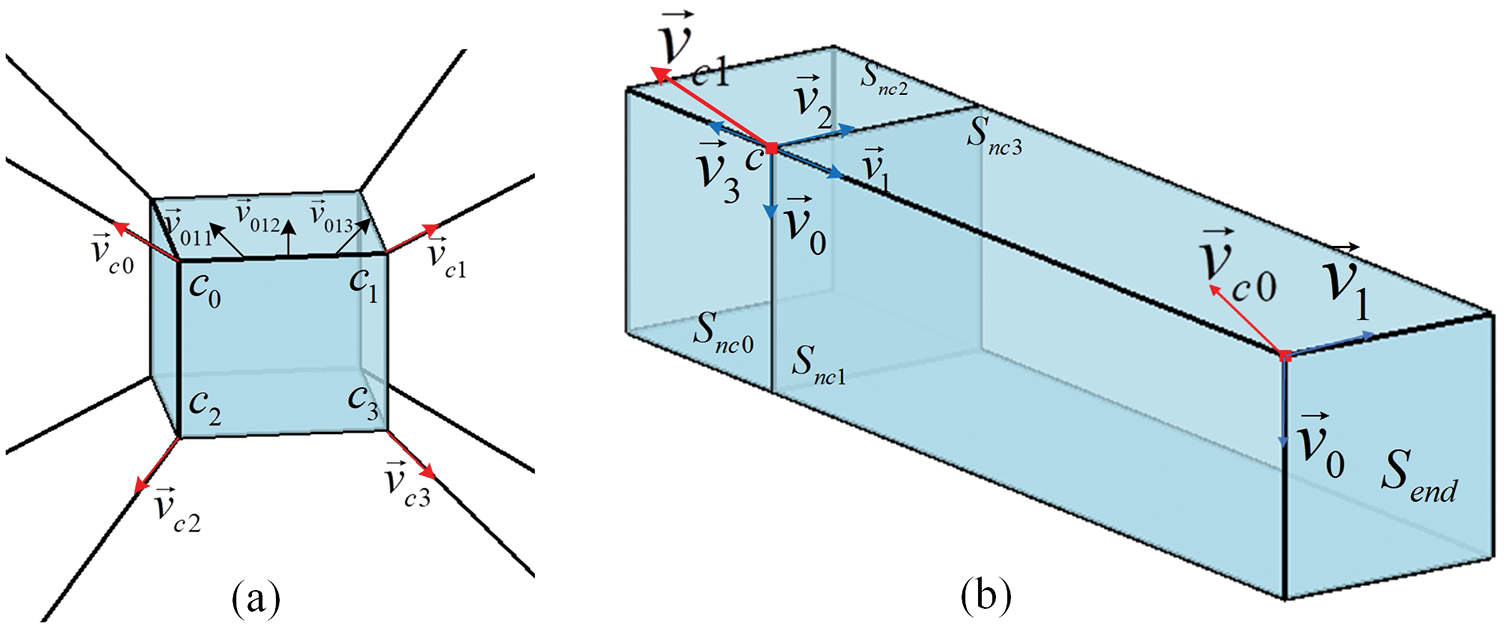Figure 8: Calculate sampling vectors and corner vectors. (a) Sampling vectors of the boundary surface; (b) calculate corner sampling vector

The vector of each corner ci is calculated according to Eq. (11).

vc=i=0mvi(11)

When the corner point ci belongs to Snc, the sampling vectorvc1=(v0+v1+v2+v3). Otherwise, the sampling vector vc0=(v0+v1), as shown in Fig. 8b.

The sampling vector on the edge Ci is calculated according to Eq. (12). Ci(uk) is uniformly distributed on the edge Ci, where K is the number of points. Rotate(vR,vP,α) represents the counterclockwise rotation of vPby an angle α on the axis vR. θ is the angle between vP and vQ.

vk=Ci(uk)+Rotate(vR,vP,α)(k=0,,K1)uk=k/(K1)vR=vP×vQα=θuk(12)

The Newton iteration method is used to find the intersection points on the transition surface. The sampling points and the Coons interpolation algorithm are used to fit the B-spline curves Ci(u)(i=0,1,2,3). With the four boundary curves C0,C1,C2, and C3, the B-spline surface S(u,v) is constructed by interpolation, as shown in Fig. 9a.Figure 9: Outer hexahedron group modeling. (a) Boundary surface and transition surface mapping; (b) outer hexahedron group

According to the mapping between Snc and S(u,v), the volume interpolation algorithm  is used to construct the outer hexahedron group Vouters. The volume parametric node VBn is expressed by Eq. (13).

VBn=Vinters+Vouters(13)

The local patches of the outer hexahedron group and the final volume parametric node are shown in Fig. 9b.

4.3 Modeling of Joint Nodes and End Nodes

After completing the modeling of the branch node, the cross-section is obtained, as shown in Fig. 4a. Each cross-section is expressed by Eq. (14).

S(u,v)=i=0nj=0mNi,p(u)Nj,q(v)ωi,jPi,jSi=0nj=0mNi,p(u)Nj,q(v)ωi,j(14)

The end node En is constructed with the cross-section group SEn=(S0,S1,S2,S3,S4) and the skeleton curve CEn, as expressed in Eq. (15).

CEn(w)=k=0lNk,r(w)ωkPkLk=0lNk,r(w)ωk(15)

The stretch volumes are constructed through affine transformations of the section group along the skeleton curve as shown in Fig. 10a.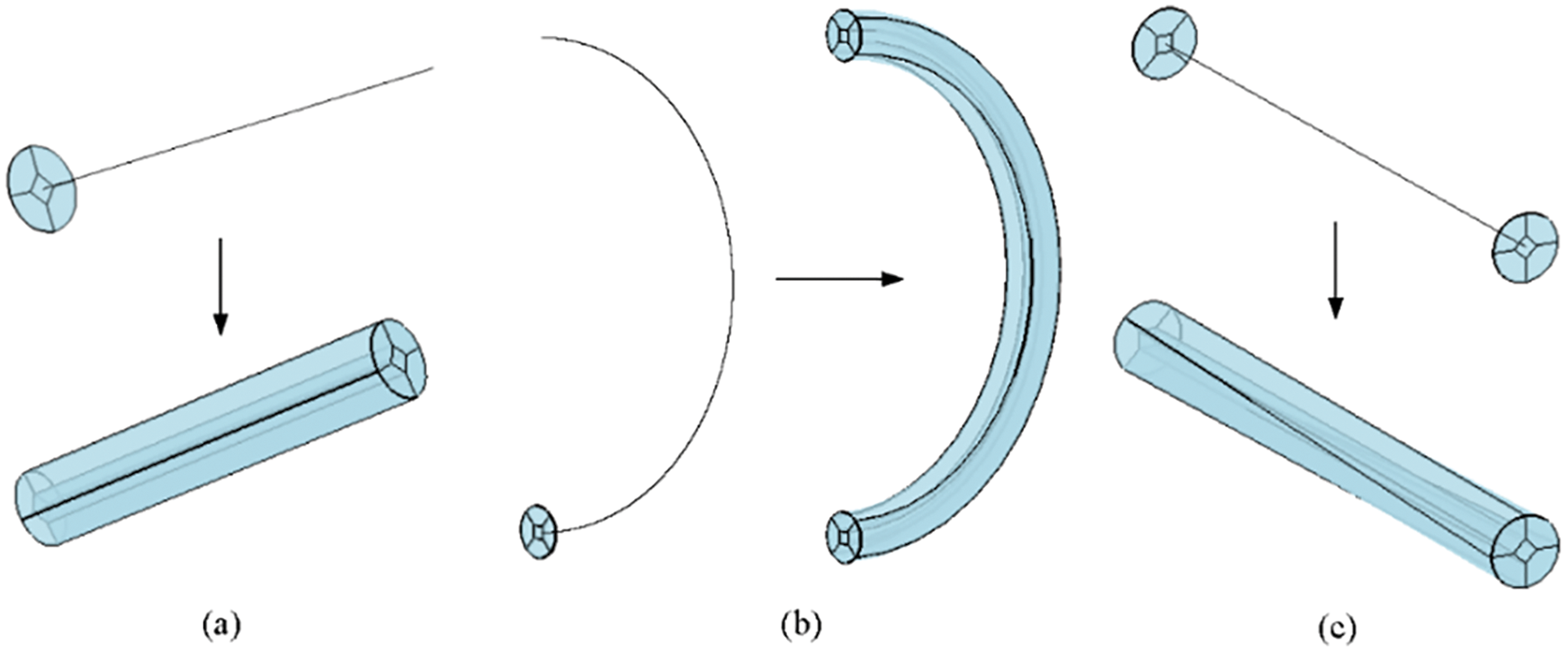Figure 10: Node modeling. (a) Extruding; (b) sweeping; (c) lofting

In addition, sweeping is another method to construct nodes. Both SEn(u,v),C(ωk) and the number of section groups k are needed. The number of knots Nω=r+k+2. r is the degree of the parameter ω. The value of ωk depends on the knot vector W. The Frenet frame of the cross-section is constructed according to the knot, as shown in Eq. (16). The matrix of the control vertices is obtained by Eq. (17).

F0k=C(wk)F1k=C(wk)×C(wk)|C(wk)×C(wk)|F2k=F1i×C(wk)F3k=C(wk)(16)

PN=QN=[ N0,r(w0)N1,r(w0)Nr,r(w0)N1,r(w1)N2,r(w1)Nr+1,r(w1)Nir,r(wK)Nir+1,r(wK)Nk,r(wK) ]                          Q=[ Q0Q1Qi ]                Qi=[ Qi,0,0Qi,1,0Qi,j,0Qi,0,1Qi,1,1Qi,j,1Qi,0,kQi,1,kQi,j,k ](17)

The other control points Qi,j,k are calculated with the projection of the section group SEn(u,v), as shown in Eq. (18).

Qi,j,k=ωi,jM1M2Pi,jS(18)

Here, ω is the weight of the control point PSi,j. M1 is the transformation matrix between the local and the global coordinate system, M2 is the Frenet transformation matrix. The construction of sweeping is shown in Fig. 10b.

The joint node is constructed with the two section groups SJn1=(S0,S1,S2,S3,S4) and SJn2=(S0,S1,S2,S3,S4) along the skeleton curve CJn(ω). Multiple section groups are calculated by interpolation, which is similar to the sweeping method. The joint node is shown in Fig. 10c.

5  Modeling of the Unit Cell and the Lattice Structure

5.1 Modeling of the Unit Cell

The unit-cell of the lattice structure describes the local characteristics. The geometric parameters that control the specific shape of the unit-cell mainly include the size (i.e., length, width, and height), section radius, and horizontal angles. According to the geometry and topology of the skeleton model, a volume parametric unit-cell is constructed through translation, rotation, and reflection of the nodes. The relationship between the unit-cell Vuc(u,v,w) and the skeleton model Cuc(u) is expressed by Eq. (19).

Gs=(Vuc(u,v,w),Cuc(u))(19)

For example, the skeleton of the simple cubic unit-cell shown in Fig. 11c is split into two basic nodes, as shown in Figs. 11a and 11b. The volume parametric models of the two nodes are constructed as illustrated in Figs. 11d and 11e. The final unit-cell model is obtained through translation, rotation, and splicing operations, as shown in Fig. 12f.Figure 11: Simple cubic unit-cell model generation. (a) Node skeleton; (b) node skeleton; (c) unit-cell skeleton; (d) node model; (e) node model; (f) unit-cell model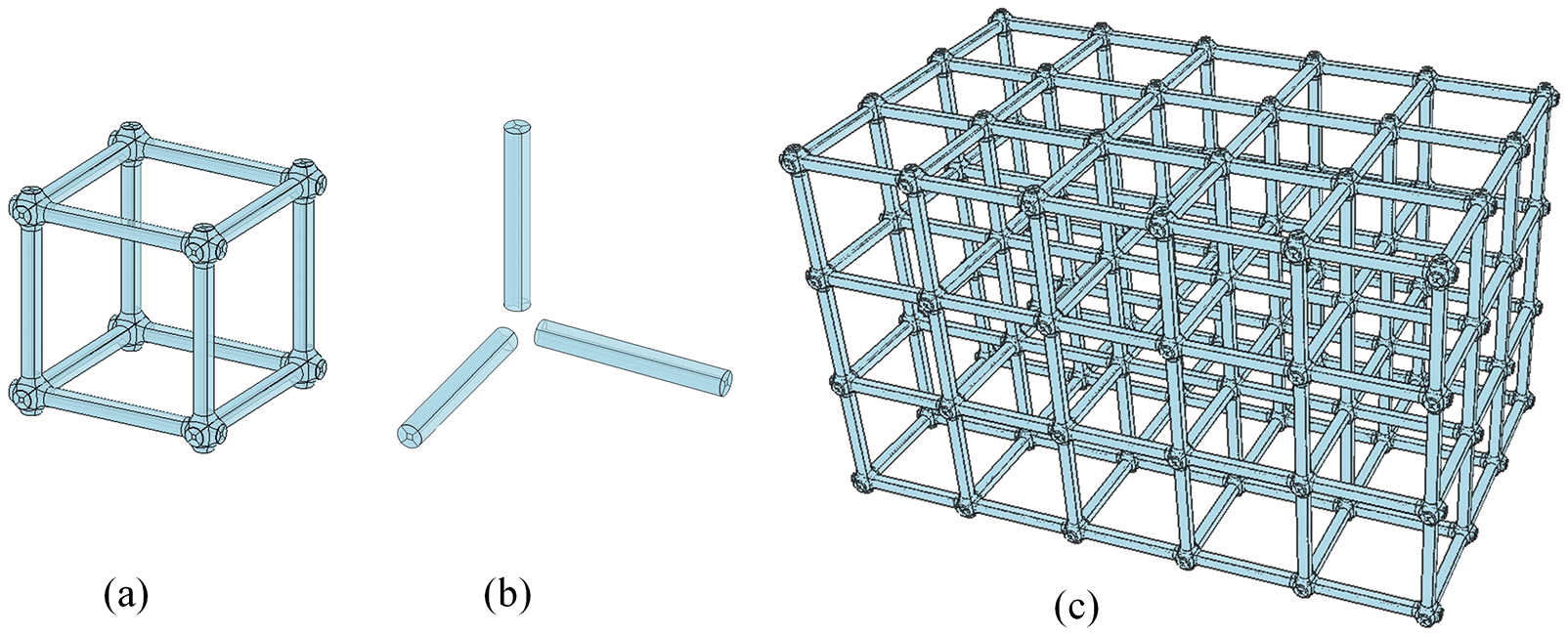Figure 12: Simple cubic lattice structure model generation. (a) Unit-cell model; (b) joint node model; (c) lattice structure model

5.2 Modeling of the Lattice Structure

The unit-cell of the uniform lattice structure is a parallelepiped. Three translation basis vectors intersect at the vertex. The skeleton with three orthogonal translation basis vectors is called the cubic lattice structure. Due to the unique symmetry properties, the cubic lattice structure is the most widely used type. The modeling process mainly includes two steps. Firstly, the unit-cell is constructed as the basic element. Secondly, duplicate the unit-cells in space to form the lattice structure. The center of the unit-cell is used to indicate the location. Finally, unit-cells are arranged along the translation basis vectors to generate the volume parametric lattice structures.

A simple cubic lattice structure that satisfies geometric connectivity is shown in Fig.12. The volume parametric unit-cell is composed of 308 trivariate NURBS volumes as shown in Fig. 12a. We set the translation basis vectors as the coordinate axis directions, and a series of operations involving the translation basis vector directions are executed. The connectivity among unit-cells is guaranteed by the joint node as shown in Fig. 12b. The volume parametric lattice structure with 4096 trivariate NURBS volumes is constructed as shown in Fig. 12c.

6  Examples and Simulation

We constructed several models of node and lattice structure to prove the effectiveness and applicability of the proposed method. In the meantime, the Jacobian values of the models are calculated and visualized to evaluate the quality. And the IGA is utilized to verify the feasibility of integration of modeling and simulation of our method.

6.1 Examples of Branch Node Models

Several nodes are constructed with the modeling method presented in Section 4. The nodes with orthogonal branches are shown in Fig. 13, and the others are shown in Fig. 14. The branch curves with the hexahedron boxes are shown in column (a), the inner hexahedron groups are shown in column (b), the transition surface models constructed with multiple toric surfaces are shown in column (c), and the volume parametric nodes are shown in column (d). The parameters of nodes are listed in Table 1. The first four cases are classified as orthogonal nodes, the length of the branch curves is 2, and the diameter of the cross-section is 2. The last five cases are classified as complex nodes with a length of 3 and the same diameter.Figure 13: Modeling process of orthogonal branch nodes. (a) Hexahedron box; (b) inner hexahedron groups; (c) transition surfaces; (d) volumetric parameterization nodesFigure 14: Modeling process of complex branch nodes. (a) Hexahedron box; (b) inner hexahedron groups; (c) transition surfaces; (d) volumetric parameterization nodes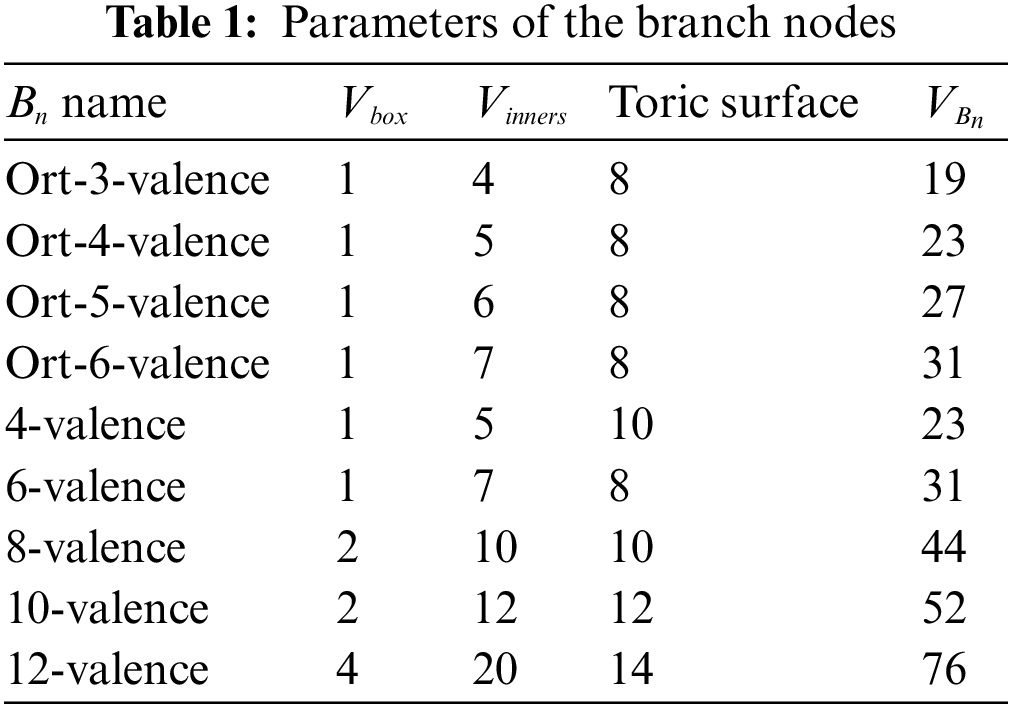6.2 Examples of Unit-Cell Models

According to Section 5, we construct some typical unit-cell models as shown in Fig.15. The parameters are listed in Table 2. The diameter of the cross-section of joint nodes is 2.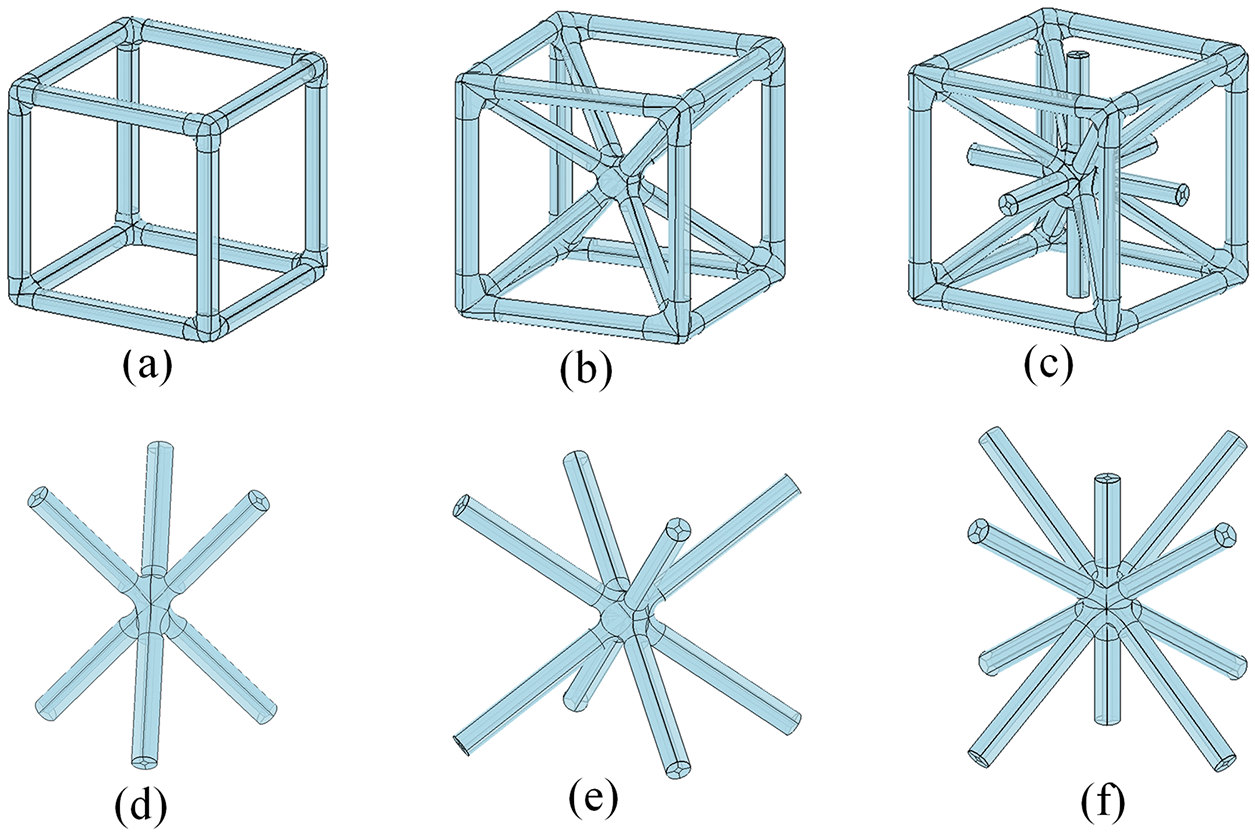Figure 15: The unit-cell models. (a) Simple cubic unit-cell(Cell-1); (b) body-centered cubic unit-cell(Cell-2); (c) face-body-centered cubic unit-cell(Cell-3); (d) 3D-Kagome unit-cell(Cell-4); (e) 3D-pyramidal unit-cell(Cell-5); (f) body-centered-plus unit-cell(Cell-6)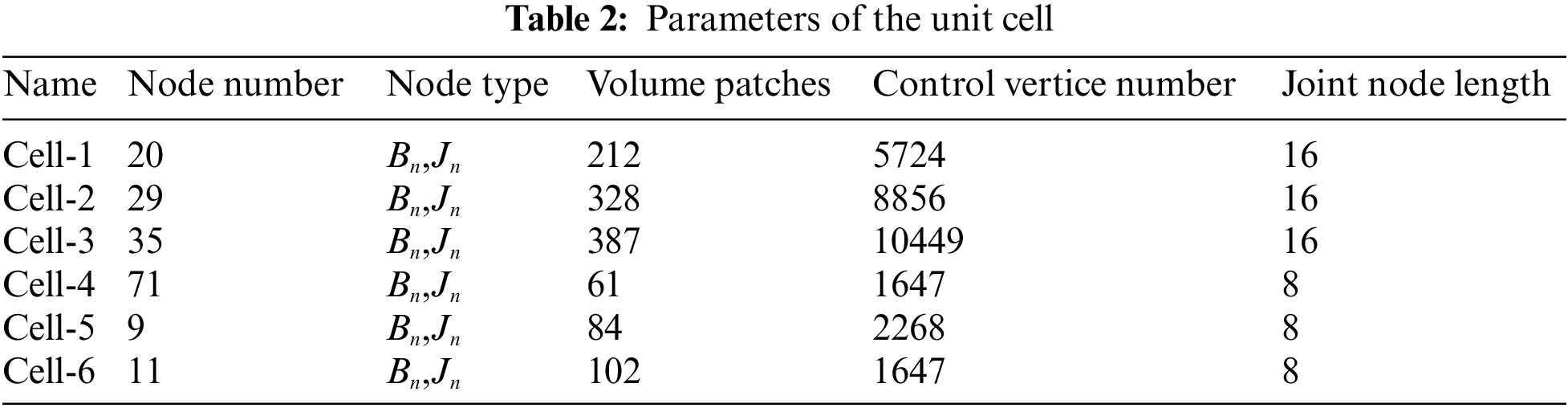6.3 Examples of Lattice Structures

Based on the abovementioned unit-cells, several different types of volume parametric lattice structures are constructed as shown in Fig. 16. Some sandwich-type of lattice structures are also built as shown in Fig. 17. The 3D view of the models is presented in the first column, and the front view is illustrated in the second column. The parameters of the lattice structures are listed in Table 3.Figure 16: Multiple cubic lattice structure models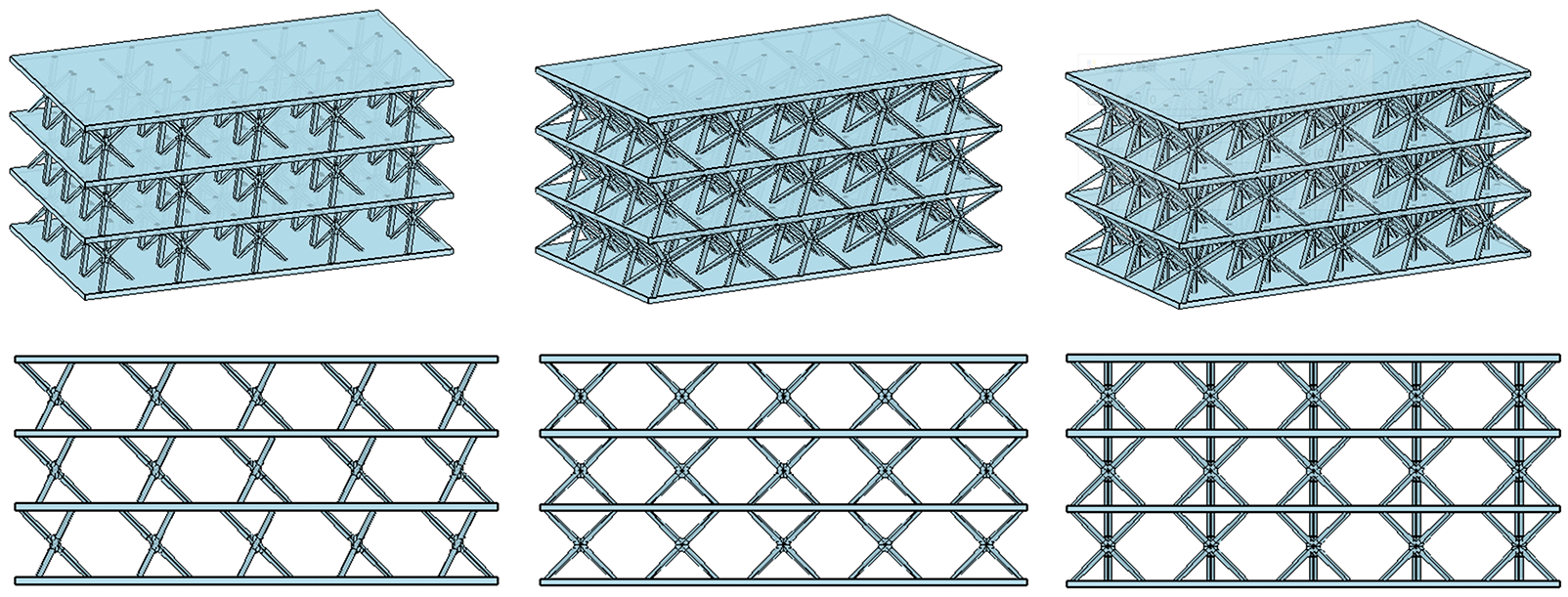Figure 17: Sandwich-type lattice structure models6.4 Evaluation of the Model Quality

To verify the volume parametric model is suitable for IGA without intersections, overlaps, or large-angle distortions of hexahedrons, the value of the Jacobian matrix is the main index for quality assessment . In addition, the Jacobian value should be greater than zero and the distribution should be as evenly as possible within the parameter domain. The calculation of the Jacobian value is provided with the expression Eq. (20).

J=|VuVvVw|=|xuxvxwyuyvywzuzvzw|(20)

The Jacobian values of the nodes and the unit cells are shown in Figs. 1820. The Jacobian values are bigger than zero, and the distribution is relatively symmetrical. The quality evaluation of volume parametric lattice structures is shown in Fig. 21. The Jacobian values demonstrate excellent quality and satisfy the requirement of analysis. Therefore, the quality of the models obtained by the proposed method is verified.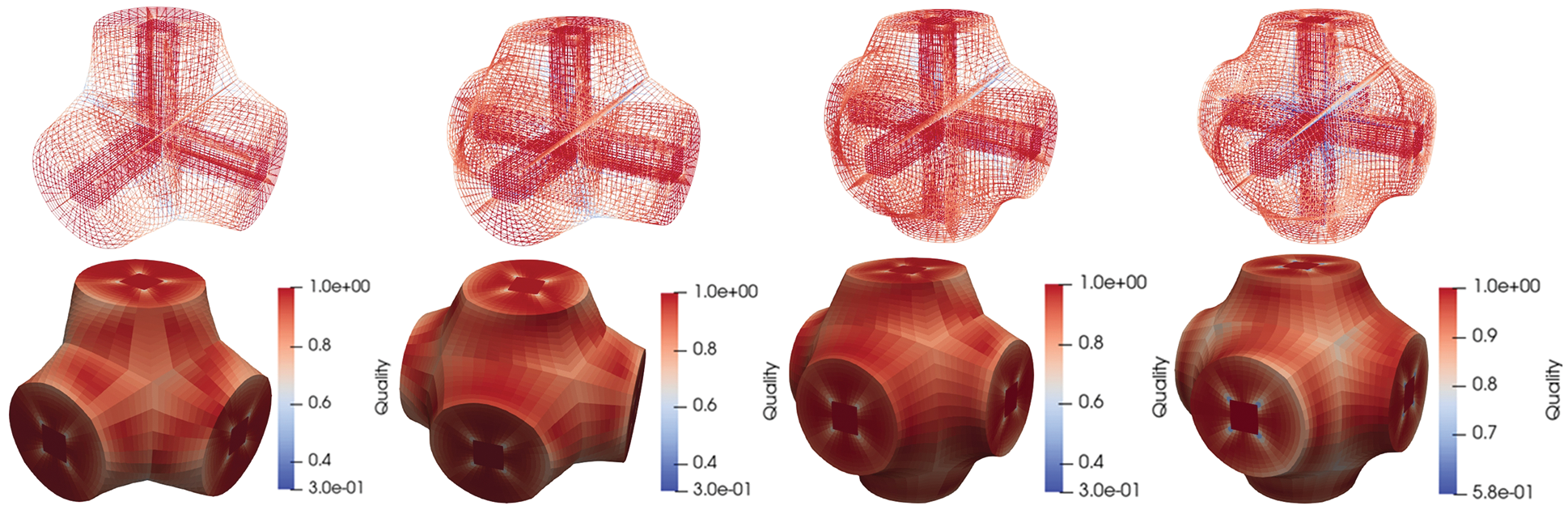Figure 18: Jacobian values of orthogonal branch nodes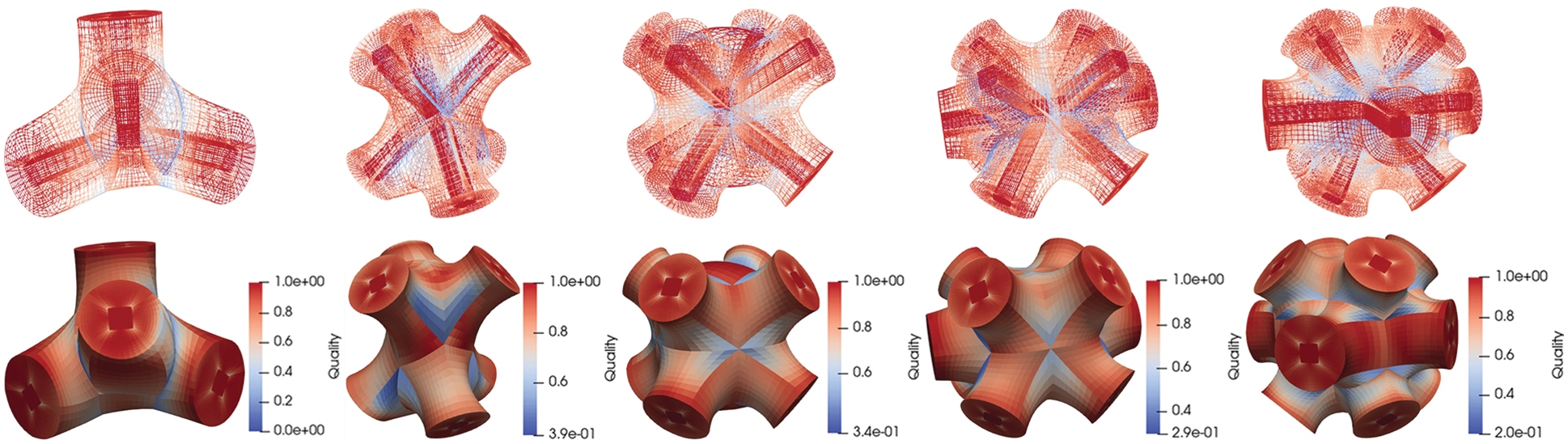Figure 19: Jacobian values of complex branch nodesFigure 20: Jacobian values of unit-cell models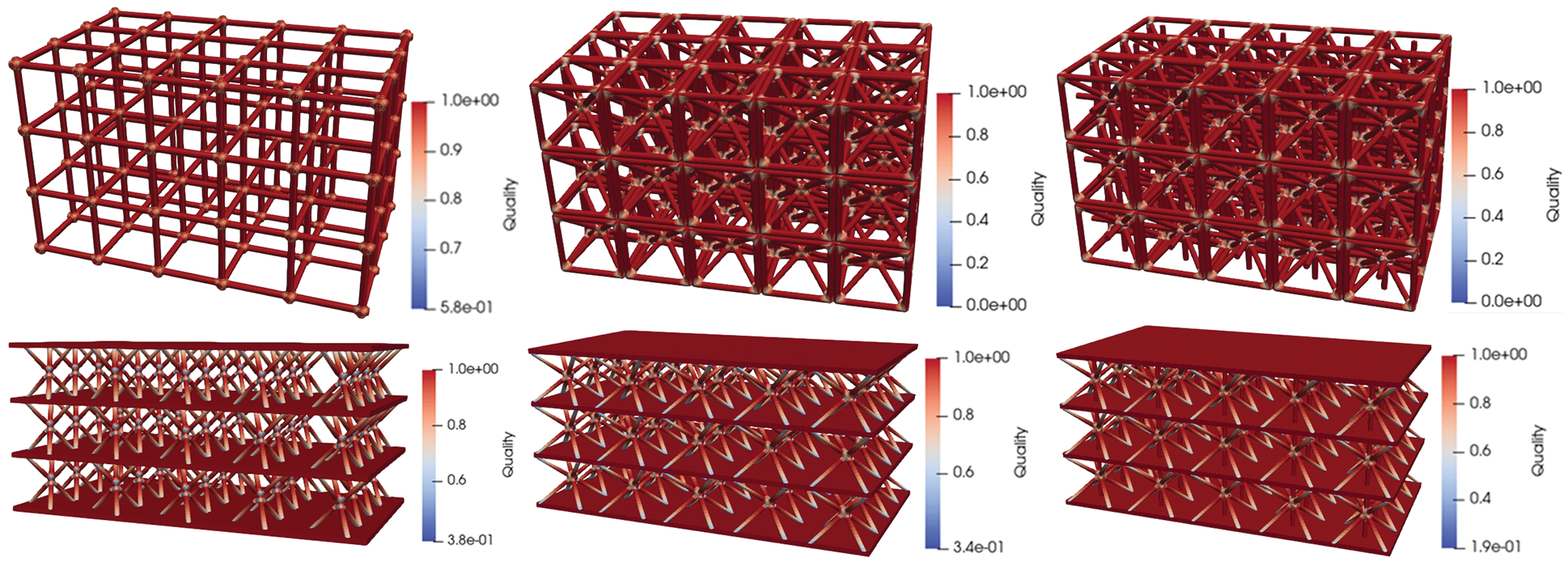Figure 21: Jacobian values of the lattice structure models

There are some singularities in the models constructed by the proposed method, which are reflected in the minimum values of the Jacobian. Singularities damage the quality of the model. How to control the singularity is a major issue of geometric modeling. Researchers have proposed some approaches, such as utilizing sparse distributed directional constraints to determine the appropriate singularities , designing different templates to handle the features of new singularities , or using a practical framework to adjust singularity graphs by modifying the rotational transition . Some researchers use novel tetrahedral split operations to preprocess singularity-restricted frame fields . These methods achieve the goal of controlling the singularity but bring a huge extra computation at the same time. The model of lattice structure is especially difficult to deal with singularities with complex nodes and massive volume parametric patches. Considering the Jacobian values still meet the requirement of IGA, the singularities are not specifically controlled in the modeling process of this paper.

6.5 Model Verification via IGA

We applicated IGA to the volume parametric models to verify the applicability. As shown in Figs. 22 and 23, the boundary conditions are shown in column (a). We set the material as stainless steel, Young’s modulus is 2.0×1011 Pa, Poisson’s ratio is 0.3, Bulk modulus is 1.6667×1011 Pa, and Shear modulus is 7.6923×1010 Pa. The results of IGA are shown in column (b). We added the FEA results of the same geometric model as a comparison in column (c).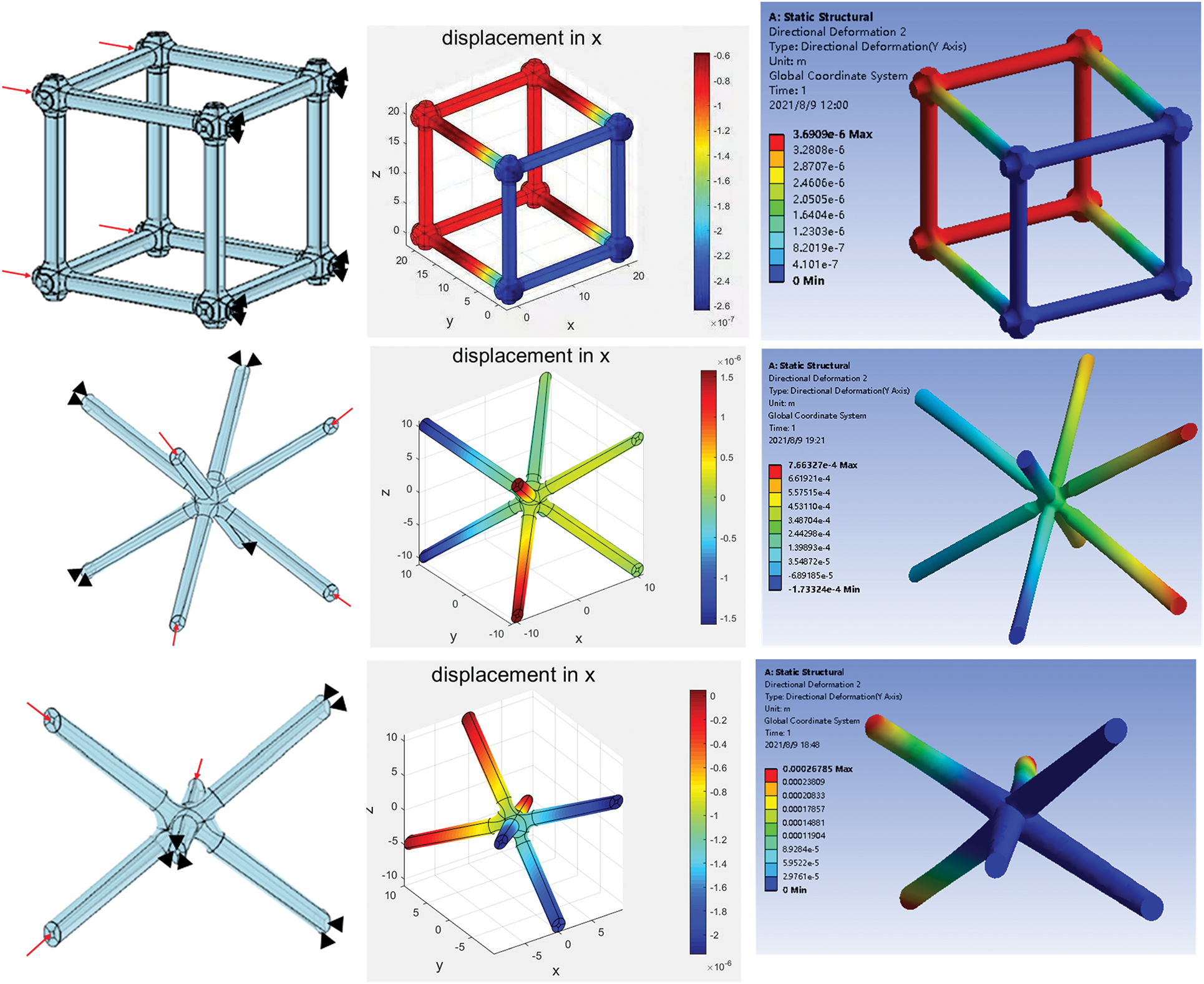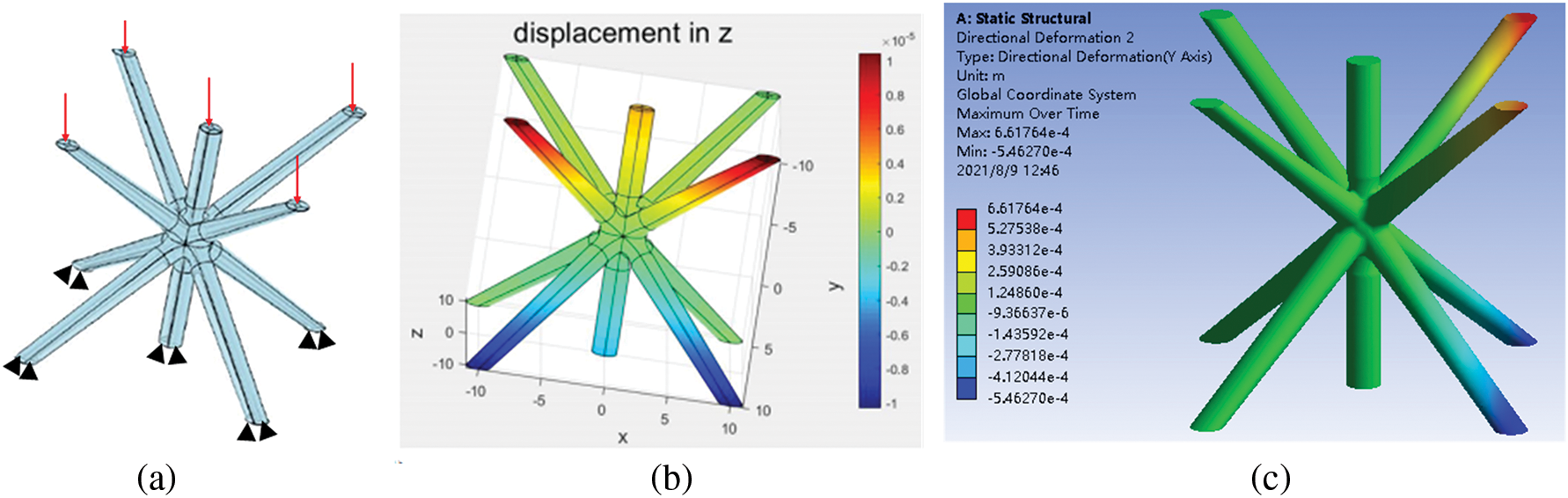Figure 22: Results of the IGA and FEA conducted on the unit-cell models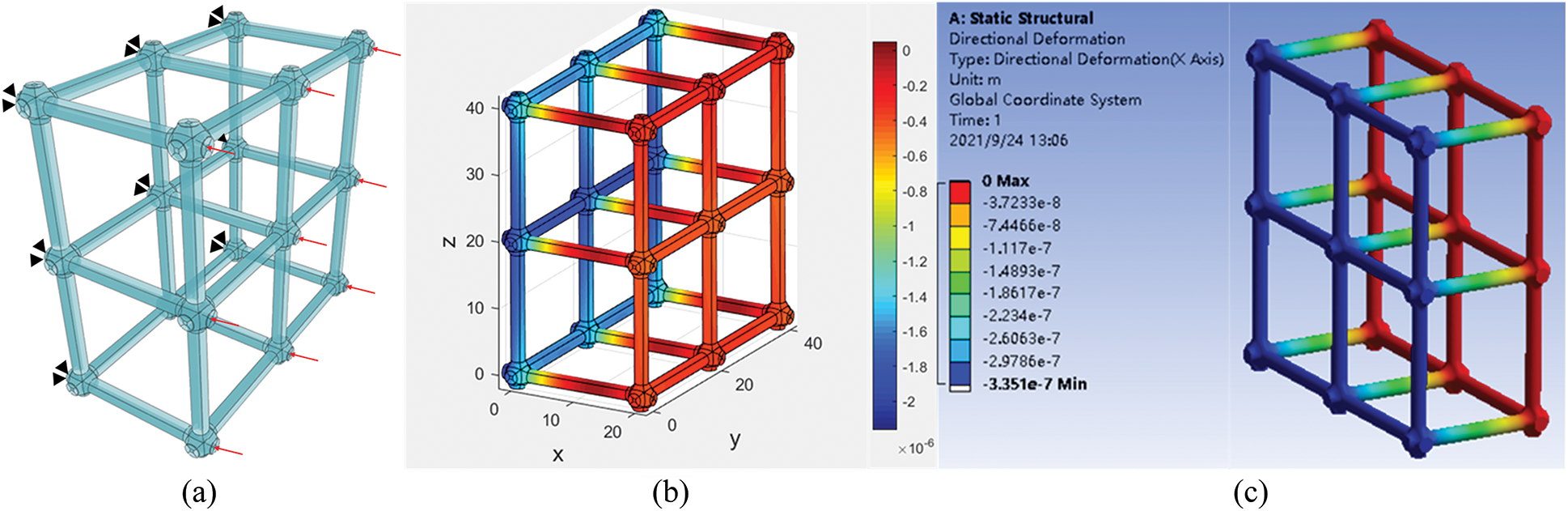Figure 23: Results of the IGA and FEA conducted on the lattice structure model

The boundary conditions are illustrated in Fig.22a. The load is equal to 440N, and the adaptive mesh numbers of the four cases are 36445, 7438, 5545, and 2672, respectively. The maximum displacements of the four models are 2.6×107, 2.5×106, 1.5×106, and 1.1×105 mm. The results of FEA calculated by commercial software are similar, and the distribution of values is the same. The IGA is also applicated to a simple lattice structure as shown in Fig. 23. We can see the results of the two methods exhibit the same distribution as shown in Figs. 23b and 23c.

With the above examples, we have proved the volume parametric models constructed by our method are suitable for IGA, and the feasibility of integration of modeling and simulation is verified too.

7  Conclusions

In this paper, a volume parametric modeling method of 3D lattice structures based on the skeleton model is proposed. The unit-cell is combined with different volume parametric nodes. And the volume parametric lattice structure is assembled with the periodic arrangements of the unit-cells. The effectiveness and stability of the proposed method are proved with numerous examples. The quality of the volume parametric models is evaluated by Jacobian values. And finally, the feasibility of integration of modeling and simulation is proved with isogeometric analysis.

However, the quality of complex nodes is still not perfect enough, and how to control the singularity is also an important issue. In addition, the manufacturing of volume parametric lattice structures is worth to be studied in the future, and applying these models to crystal dynamics is also significant.

Funding Statement: This research is supported by the National Nature Science Foundation of China under Grant No. 52075340.

Conflicts of Interest: The authors declare that they have no conflicts of interest to report regarding the present study.

References

1.  1.  Chu, C., Graf, G., Rosen, D. W. (2008). Design for additive manufacturing of cellular structures. Computer-Aided Design and Applications, 5(5), 686–696. DOI 10.3722/cadaps.2008.686-696.
2.  2.  Schmelzle, J., Kline, E. V., Dickman, C. J., Reutzel, E. W., Jones, G. et al. (2015). (Re) Designing for part consolidation: Understanding the challenges of metal additive manufacturing. Journal of Mechanical Design, 137(11), 111404. DOI 10.1115/1.4031156.
3.  3.  Hutchinson, J. W., Xue, Z. (2005). Metal sandwich plates optimized for pressure impulses. International Journal of Mechanical Sciences, 47(4–5), 545–569. DOI 10.1016/j.ijmecsci.2004.10.012.
4.  4.  Liu, T., Deng, Z. C., Lu, T. J. (2007). Bi-functional optimization of actively cooled, pressurized hollow sandwich cylinders with prismatic cores. Journal of the Mechanics and Physics of Solids, 55(12), 2565–2602. DOI 10.1016/j.jmps.2007.04.007.
5.  5.  Vasiliev, V. V., Razin, A. F. (2006). Anisogrid composite lattice structures for spacecraft and aircraft applications. Composite Structures, 76(1–2), 182–189. DOI 10.1016/j.compstruct.2006.06.025.
6.  6.  Hughes, T. J. (2012). The finite element method: Linear static and dynamic finite element analysis. Chelmsford: Courier Corporation.
7.  7.  Lei, H., Li, C., Meng, J., Zhou, H., Liu, Y. et al. (2019). Evaluation of compressive properties of SLM-fabricated multi-layer lattice structures by experimental test and μ-CT-based finite element analysis. Materials & Design, 169(6058), 107685. DOI 10.1016/j.matdes.2019.107685.
8.  8.  Cottrell, J. A., Hughes, T. J., Bazilevs, Y. (2009). Isogeometric analysis: Toward integration of CAD and FEA. Hoboken: John Wiley & Sons.
9.  9.  Hughes, T. J., Cottrell, J. A., Bazilevs, Y. (2005). Isogeometric analysis: CAD, finite elements, NURBS, exact geometry and mesh refinement. Computer Methods in Applied Mechanics and Engineering, 194(39–41), 4135–4195. DOI 10.1016/j.cma.2004.10.008.
10. 10. Massarwi, F., Elber, G. (2016). A B-spline based framework for volumetric object modeling. Computer-Aided Design, 78(5526), 36–47. DOI 10.1016/j.cad.2016.05.003.
11. 11. Fan, H. L., Jin, F. N., Fang, D. N. (2008). Mechanical properties of hierarchical cellular materials. Part I: Analysis. Composites Science and Technology, 68(15–16), 3380–3387. DOI 10.1016/j.compscitech.2008.09.022.
12. 12. Masalha, R., Cirillo, E., Elber, G. (2021). Heterogeneous parametric trivariate fillets. Computer Aided Geometric Design, 86, 101970. DOI 10.1016/j.cagd.2021.101970.
13. 13. Li, J., Zheng, W., Su, Y., Hong, F. (2022). Pore scale study on capillary pumping process in three-dimensional heterogeneous porous wicks using Lattice Boltzmann method. International Journal of Thermal Sciences, 171, 107236. DOI 10.1016/j.ijthermalsci.2021.107236.
14. 14. Leblanc, L., Houle, J., Poulin, P. (2011). Modeling with blocks. The Visual Computer, 27(6), 555–563. DOI 10.1007/s00371-011-0589-4.
15. 15. Tang, Y., Kurtz, A., Zhao, Y. F. (2015). Bidirectional evolutionary structural optimization (BESO) based design method for lattice structure to be fabricated by additive manufacturing. Computer-Aided Design, 69, 91–101. DOI 10.1016/j.cad.2015.06.001.
16. 16. Tang, Y., Dong, G., Zhao, Y. F. (2019). A hybrid geometric modeling method for lattice structures fabricated by additive manufacturing. The International Journal of Advanced Manufacturing Technology, 102(9), 4011–4030. DOI 10.1007/s00170-019-03308-x.
17. 17. Liu, S., Liu, T., Zou, Q., Wang, W., Doubrovski, E. L. et al. (2021). Memory-efficient modeling and slicing of large-scale adaptive lattice structures. Journal of Computing and Information Science in Engineering, 21(6), 061003. DOI 10.1115/1.4050290.
18. 18. Li, S., Ren, B., Minaki, H. (2014). Multiscale crystal defect dynamics: A dual-lattice process zone model. Philosophical Magazine, 94(13), 1414–1450. DOI 10.1080/14786435.2014.887859.
19. 19. Lyu, D., Li, S. (2017). Multiscale crystal defect dynamics: A coarse-grained lattice defect model based on crystal microstructure. Journal of the Mechanics and Physics of Solids, 107(3), 379–410. DOI 10.1016/j.jmps.2017.07.006.
20. 20. Lyu, D., Li, S. (2019). A multiscale dislocation pattern dynamic: Towards an atomistic-informed crystal plasticity theory. Journal of the Mechanics and Physics of Solids, 122(3), 613–632. DOI 10.1016/j.jmps.2018.09.025.
21. 21. Zhang, L. W., Xie, Y., Lyu, D., Li, S. (2019). Multiscale modeling of dislocation patterns and simulation of nanoscale plasticity in body-centered cubic (BCC) single crystals. Journal of the Mechanics and Physics of Solids, 130(170), 297–319. DOI 10.1016/j.jmps.2019.06.006.
22. 22. Xie, Y., Li, S. (2021). Geometrically-compatible dislocation pattern and modeling of crystal plasticity in body-centered cubic (BCC) crystal at micron scale. Computer Modeling in Engineering & Sciences, 129(3), 1419–1440. DOI 10.32604/cmes.2021.016756.
23. 23. Dong, G., Tang, Y., Zhao, Y. F. (2017). A survey of modeling of lattice structures fabricated by additive manufacturing. Journal of Mechanical Design, 139(10), 100906. DOI 10.1115/1.4037305.
24. 24. Medeiros e Sa, A., Mello, V. M., Rodriguez, E. K., Covill, D. (2015). Adaptive voids. The Visual Computer, 31(6), 799–808. DOI 10.1007/s00371-015-1109-8.
25. 25. Tang, Y., Dong, G., Zhou, Q., Zhao, Y. F. (2017). Lattice structure design and optimization with additive manufacturing constraints. IEEE Transactions on Automation Science and Engineering, 15(4), 1546–1562. DOI 10.1109/TASE.2017.2685643.
26. 26. Garner, E., Kolken, H. M., Wang, C. C., Zadpoor, A. A., Wu, J. (2019). Compatibility in microstructural optimization for additive manufacturing. Additive Manufacturing, 26(1), 65–75. DOI 10.1016/j.addma.2018.12.007.
27. 27. Conde-Rodríguez, F., Torres, J. C., García-Fernández, Á. L., Feito-Higueruela, F. R. (2017). A comprehensive framework for modeling heterogeneous objects. The Visual Computer, 33(1), 17–31. DOI 10.1007/s00371-015-1149-0.
28. 28. Xu, G., Mourrain, B., Duvigneau, R., Galligo, A. (2013). Analysis-suitable volume parameterization of multi-block computational domain in isogeometric applications. Computer-Aided Design, 45(2), 395–404. DOI 10.1016/j.cad.2012.10.022.
29. 29. Hsu, M. C., Wang, C., Herrema, A. J., Schillinger, D., Ghoshal, A. et al. (2015). An interactive geometry modeling and parametric design platform for isogeometric analysis. Computers & Mathematics with Applications, 70(7), 1481–1500. DOI 10.1016/j.camwa.2015.04.002.
30. 30. Liang, Y., Zhao, F., Yoo, D. J., Zheng, B. (2020). Design of conformal lattice structures using the volumetric distance field based on parametric solid models. Rapid Prototyping Journal, 26(6), 1005–1017. DOI 10.1108/RPJ-04-2019-0114.
31. 31. Livesu, M., Muntoni, A., Puppo, E., Scateni, R. (2016). Skeleton-driven adaptive hexahedral meshing of tubular shapes. Computer Graphics Forum, 35(7), 237–246. DOI 10.1111/cgf.13021.
32. 32. Xu, G., Kwok, T. H., Wang, C. C. (2017). Isogeometric computation reuse method for complex objects with topology-consistent volumetric parameterization. Computer-Aided Design, 91(39–41), 1–13. DOI 10.1016/j.cad.2017.04.002.
33. 33. Elber, G., Kim, Y. J., Kim, M. S. (2012). Volumetric boolean sum. Computer Aided Geometric Design, 29(7), 532–540. DOI 10.1016/j.cagd.2012.03.003.
34. 34. Xiao, X., Sabin, M., Cirak, F. (2019). Interrogation of spline surfaces with application to isogeometric design and analysis of lattice-skin structures. Computer Methods in Applied Mechanics and Engineering, 351, 928–950. DOI 10.1016/j.cma.2019.03.046.
35. 35. Li, K., Wu, D., Chen, X., Cheng, J., Liu, Z. et al. (2018). Isogeometric analysis of functionally graded porous plates reinforced by graphene platelets. Composite Structures, 204(6), 114–130. DOI 10.1016/j.compstruct.2018.07.059.
36. 36. Yu, Y. Y., Ji, Y., Zhu, C. G. (2020). An improved algorithm for checking the injectivity of 2D toric surface patches. Computers & Mathematics with Applications, 79(10), 2973–2986. DOI 10.1016/j.camwa.2020.01.001.
37. 37. Li, J., Ji, Y., Zhu, C. (2021). De casteljau algorithm and degree elevation of toric surface patches. Journal of Systems Science and Complexity, 34(1), 21–46. DOI 10.1007/s11424-020-9370-y.
38. 38. Piegl, L., Tiller, W. (1996). The NURBS book. Berlin: Springer Science & Business Media.
39. 39. Tagliasacchi, A., Zhang, H., Cohen-Or, D. (2009). Curve skeleton extraction from incomplete point cloud. Special Interest Group on Computer Graphics and Interactive Techniques Conference, pp. 1–9. DOI 10.1145/1576246.
40. 40. Dey, T. K., Sun, J. (2006). Defining and computing curve-skeletons with medial geodesic function. Symposium on Geometry Processing, 6, 143–152. DOI 10.1145/1281957.1281975.
41. 41. Cao, J., Tagliasacchi, A., Olson, M., Zhang, H., Su, Z. (2010). Point cloud skeletons via laplacian based contraction. 2010 Shape Modeling International Conference, pp. 187–197. Aix-en-Provence, France.
42. 42. Tagliasacchi, A., Alhashim, I., Olson, M., Zhang, H. (2012). Mean curvature skeletons. Computer Graphics Forum, 31(5), 1735–1744. DOI 10.1111/j.1467-8659.2012.03178.x.
43. 43. Li, S., Yoshida, S., Arihara, K., Nakashima, K., Peng, Y. et al. (2021). Skeleton-based interactive fabrication for large-scale newspaper sculpture. 2021 Nicograph International (NicoInt), pp. 74–81. Tokyo.
44. 44. Soprunov, I., Soprunova, J. (2009). Toric surface codes and minkowski length of polygons. SIAM Journal on Discrete Mathematics, 23(1), 384–400. DOI 10.1137/080716554.
45. 45. Zhu, X., Ji, Y., Zhu, C., Hu, P., Ma, Z. D. (2020). Isogeometric analysis for trimmed CAD surfaces using multi-sided toric surface patches. Computer Aided Geometric Design, 79(3), 101847. DOI 10.1016/j.cagd.2020.101847.
46. 46. Liu, L., Zhang, Y., Liu, Y., Wang, W. (2015). Feature-preserving T-mesh construction using skeleton-based polycubes. Computer-Aided Design, 58(29–30), 162–172. DOI 10.1016/j.cad.2014.08.020.
47. 47. Xu, G., Mourrain, B., Duvigneau, R., Galligo, A. (2011). Parameterization of computational domain in isogeometric analysis: Methods and comparison. Computer Methods in Applied Mechanics and Engineering, 200(23–24), 2021–2031. DOI 10.1016/j.cma.2011.03.005.
48. 48. Gao, X., Huang, J., Xu, K., Pan, Z., Deng, Z. et al. (2017). Evaluating Hex-mesh quality metrics via correlation analysis. Computer Graphics Forum, 36(5), 105–116. DOI 10.1111/cgf.13249.
49. 49. Chen, L., Xu, G., Wang, S., Shi, Z., Huang, J. (2019). Constructing volumetric parameterization based on directed graph simplification of l1 polycube structure from complex shapes. Computer Methods in Applied Mechanics and Engineering, 351(3), 422–440. DOI 10.1016/j.cma.2019.01.036.
50. 50. Yu, P., Anitescu, C., Tomar, S., Bordas, S. P. A., Kerfriden, P. (2018). Adaptive isogeometric analysis for plate vibrations: An efficient approach of local refinement based on hierarchical a posteriori error estimation. Computer Methods in Applied Mechanics and Engineering, 342(39), 251–286. DOI 10.1016/j.cma.2018.08.010.
51. 51. Bommes, D., Zimmer, H., Kobbelt, L. (2009). Mixed-integer quadrangulation. ACM Transactions on Graphics (TOG), 28(3), 1–10.
52. 52. Jiang, T., Huang, J., Wang, Y., Tong, Y., Bao, H. (2013). Frame field singularity correctionfor automatic hexahedralization. IEEE Transactions on Visualization and Computer Graphics, 20(8), 1189–1199. DOI 10.1109/TVCG.2013.250.
53. 53. Li, Y., Liu, Y., Wang, W., Guo, B. (2012). All-hex meshing using singularity-restricted field. ACM Transactions on Graphics, 31(6), 1–11.

Chen, L., Liang, S., Yan, N., Yang, X., Li, B. (2023). Skeleton-Based Volumetric Parameterizations for Lattice Structures. CMES-Computer Modeling in Engineering & Sciences, 135(1), 687–709.This work is licensed under a Creative Commons Attribution 4.0 International License , which permits unrestricted use, distribution, and reproduction in any medium, provided the original work is properly cited.

View

• ### 1

Like

Related articles
• Zhi-Zhong Yan, Yue-Sheng Wang,...
• V.K. Tewary
• Zhi Sun, Yuxiong Yan
• Yuxi Xie, Shaofan Li
• Jun Yan, Qianqian Sui, Zhirui...